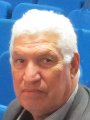Electron. J. Differential Equations, Vol. 2018 (2018), No. 130, pp. 1-27.

### Applications of the integral transforms composition method to wave-type singular differential equations and index shift transmutations Ahmed Fitouhi, Iness Jebabli, Elina L. Shishkina, Sergei M. Sitnik

Abstract:
In this article we study applications of integral transforms composition method (ITCM) for obtaining transmutations via integral transforms. It is possible to derive wide range of transmutation operators by this method. Classical integral transforms are involved in the integral transforms composition method (ITCM) as basic blocks, among them are Fourier, sine and cosine-Fourier, Hankel, Mellin, Laplace and some generalized transforms. The ITCM and transmutations obtaining by it are applied to deriving connection formulas for solutions of singular differential equations and more simple non-singular ones. We consider well-known classes of singular differential equations with Bessel operators, such as classical and generalized Euler-Poisson-Darboux equation and the generalized radiation problem of A.Weinstein. Methods of this paper are applied to more general linear partial differential equations with Bessel operators, such as multivariate Bessel-type equations, GASPT (Generalized Axially Symmetric Potential Theory) equations of Weinstein, Bessel-type generalized wave equations with variable coefficients,ultra B-hyperbolic equations and others. So with many results and examples the main conclusion of this paper is illustrated: the integral transforms composition method (ITCM) of constructing transmutations is very important and effective tool also for obtaining connection formulas and explicit representations of solutions to a wide class of singular differential equations, including ones with Bessel operators.

Submitted May 22, 2018. Published June 25, 2018.
Math Subject Classifications: 26A33, 44A15.
Key Words: Transmutations; integral transforms composition method; Bessel operator; wave-type equation; singular differential equation; Hankel transform.

Show me the PDF file (347 KB), TEX file for this article.Ahmed Fitouhi University of Tunis El Manar Tunis, Tunisia email: Ahmed.Fitouhi@fst.rnu.tn Inèss Jebabli University of Tunis El Manar Tunis, Tunisia email: jebabli.iness@hotmail.fr Elina L. Shishkina Voronezh State University Voronezh, Russia email: ilina_dico@mail.ru Sergei M. Sitnik Belgorod State National Research University (BSU) Belgorod, Russia email: sitnik@bsu.edu.ru